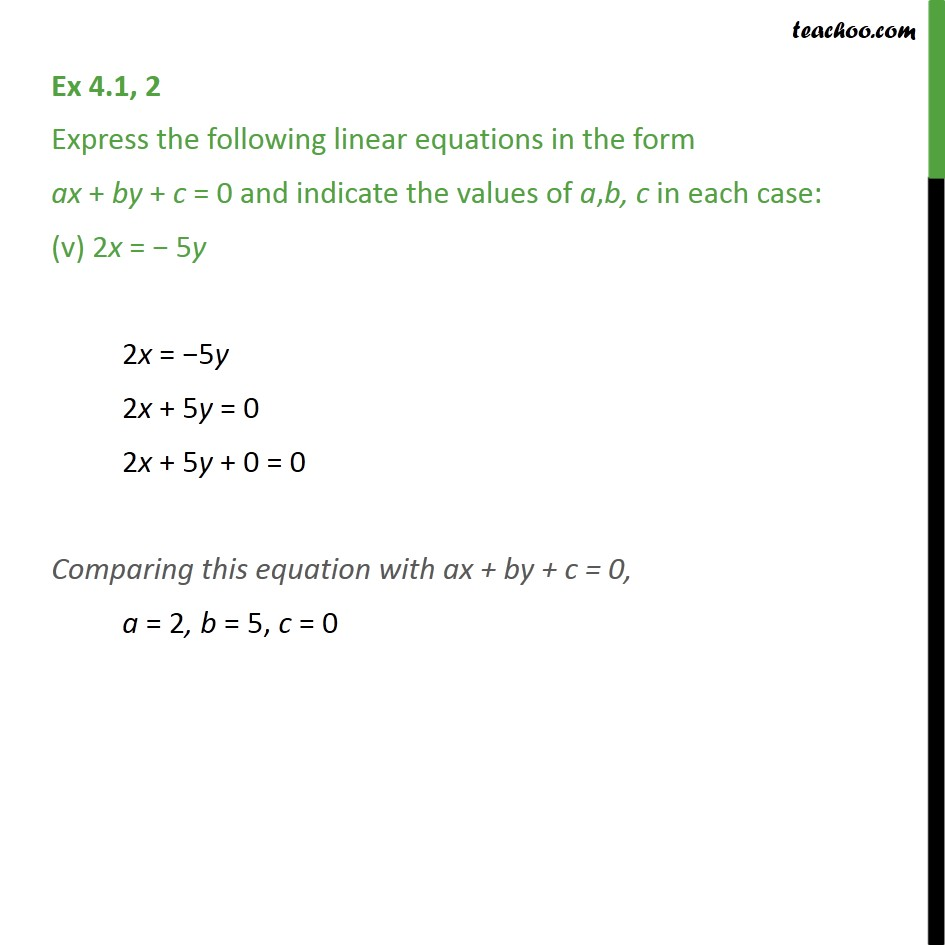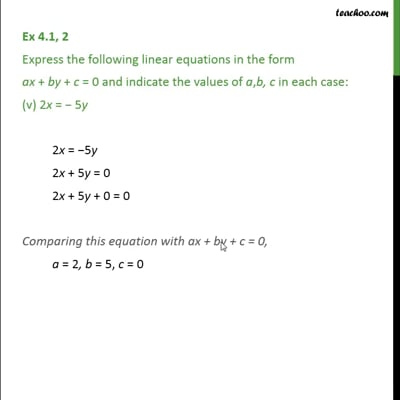Ex 4.1

Chapter 4 Class 9 Linear Equations in Two Variables
Serial order wiseThis video is only available for Teachoo black users

Introducing your new favourite teacher - Teachoo Black, at only ₹83 per month

### Transcript

Ex 4.1, 2 Express the following linear equations in the form ax + by + c = 0 and indicate the values of a,b, c in each case: (v) 2x = − 5y 2x = −5y 2x + 5y = 0 2x + 5y + 0 = 0 Comparing this equation with ax + by + c = 0, a = 2, b = 5, c = 0